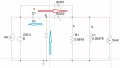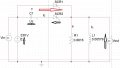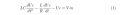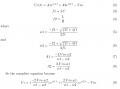# How can I estimate the value of the capacitor in my SCR extinction circuit?

#### rachidm

Joined Dec 23, 2018
4
Morning everyone, my name is Rachid and I am a student in electrical engineering. I am currently working for a school project on an extinction circuit for an SCR. The diagram can be seen below.The first diagram represent the real system. The blue arrows indicate the charging path for the capacitor. It will be charged through the diode till its voltage reaches the Input voltage value Vin. The red Arrow indicate the discharging path when SCR2is triggered. The output is a typical RL parallel load.

The SCR1 is the main SCR that feed the load and the SCR2 is used to activate the extinction circuit in order to apply a reverse voltage at the terminals of the main SCR1 during a sufficient time (in my case 200us) so that the main SCR turns off.To ease the calculations, I made the assumption that the voltage is constant during 200us as this time is smaller than the periode of the input voltage.

In transient mode, the equation which describes the behavior of the circuit during the discharge phase through the SCR is the following:

According to Kirchhoff's voltage low: Vin + Uc - Vout = 0 so Vout = Vin+Uc For current low , we have the following relation Ir + IL = Ic

At the end I got the differential equation :

** Note: at the beginning of the discharge, i. e. when the control signal is sent to SCR 2 to block SCR 1, Uc(0) = Vin and Ic(0)= 0The solution is as follow:As i've got this equation, I would like to know which efficient method i can use to determine the value of the Capacitor so that the capacitor voltage reach 0 after 200us when SCR2 is triggered.

the main problem is that the term capacity is found in all parts of the Uc equation, so it is difficult to isolate

The parameters are as follow:

-Vin = 230 V peak

-R = 0.8816 Ohm

-L = 0.00579 H

-C = ?

-tq to turn off the SCRs is 200us

Thank you for any help i can get

#### MrAl

Joined Jun 17, 2014
7,668
Hello there,

This is not that difficult of a problem but it looks like there might be some problems in the problem description and in your solution.

First, with the Vin polarity shown in the diagram, the cap can not charge up through the diode because the diode does not conduct.
That kinda stops us in our shoes right off the bat.

Second, and i am jumping ahead here, the polarities of your w1 and w2 do not look correct.

So maybe start by fixing the diagram or the description or both.

Did you design this circuit or was it handed to you?

#### rachidm

Joined Dec 23, 2018
4
Hello there,

This is not that difficult of a problem but it looks like there might be some problems in the problem description and in your solution.

First, with the Vin polarity shown in the diagram, the cap can not charge up through the diode because the diode does not conduct.
That kinda stops us in our shoes right off the bat.

Second, and i am jumping ahead here, the polarities of your w1 and w2 do not look correct.

So maybe start by fixing the diagram or the description or both.

Did you design this circuit or was it handed to you?

You're right, I made a mistake on the first schema. It should be the next one.

The voltage source must be alternative so that the capacitor can get into charging process through the diode when the input voltage became negative. When it's fully charged then there is no more current in the diode when the voltage is negative.
I built the circuit based on the methods of extinguishing the thyristor usually used.

For the polarity of w1 and w2 i got them from the auxiliary second order equation derived from the differential equation. i tried again still got the same result. So maybe i miss something.

#### MrAl

Joined Jun 17, 2014
7,668
Hi,

I had a feeling you meant that but wanted to be sure.
So the input voltage is reversed in order to charge the capacitor.
But then what happens to the input voltage after that, does it reverse back to the polarity in the drawing?
That would mean there would be the initial cap voltage and the input voltage in series, creating 2x the voltage. That's ok but not sure if you want to do that or just zero out the input voltage. So which do you want to do there?

#### crutschow

Joined Mar 14, 2008
25,122
You can't have the Vin source in parallel with the 230V source (or any two voltage sources in parallel).

#### MrAl

Joined Jun 17, 2014
7,668
You can't have the Vin source in parallel with the 230V source (or any two voltage sources in parallel).
Hi,

I took that to be a voltmeter not another voltage source.

All i need to know how is if the 230v source goes to zero after the cap charges or if it flips and goes to the polarity shown in the diagrams.

#### rachidm

Joined Dec 23, 2018
4
Hi,

I took that to be a voltmeter not another voltage source.

All i need to know how is if the 230v source goes to zero after the cap charges or if it flips and goes to the polarity shown in the diagrams.
HI,

After the cap charges, it flips and goes to the polarity shown in the diagrams.

What I want is to activate the discharge circuit while the capacitor is charged and the source voltage is positive

#### MrAl

Joined Jun 17, 2014
7,668
HI,

After the cap charges, it flips and goes to the polarity shown in the diagrams.

What I want is to activate the discharge circuit while the capacitor is charged and the source voltage is positive
Hi again,

Ok great, thanks for the info. That helps narrow down what actually has to be done.

So what you could do now is plug in the values you have already, then plug in a test value for the cap. Let's say 1uf just to start with.
Next, plot your f(t) function that you had obtained and see if the plot looks reasonable. You should see a discharging capacitor voltage that goes through zero or doesnt go through zero, but you're goal is to see it go through zero because you are asked to find the value of the cap that causes that. If it doesnt go through zero, or if it goes too soon through zero, then obviously you have to change the cap value. Once you have some approximate value you can then start to actually solve this.
Unfortunately since the cap value C is inside the formula so deeply, it may be hard to come up with a value analytically. This means you would have to use a numerical solver or use a numerical solving technique such as Newtons.
I am guessing there is no analytical solution because you are given all the other values, but i have seen miracles now and then in the past.

See if this makes sense and that you can do something with it, if not just let me know, but please try yourself first.

#### crutschow

Joined Mar 14, 2008
25,122
I took that to be a voltmeter not another voltage source.
I see that now.#### MrAl

Joined Jun 17, 2014
7,668
I see that now.Hi,

I am not used to seeing that kind of notation either and at first i couldnt figure it out, then i saw the output had the same problem and that brought it all together finallySome people like to draw meters almost like voltage sources#### rachidm

Joined Dec 23, 2018
4
Hi again,

Ok great, thanks for the info. That helps narrow down what actually has to be done.

So what you could do now is plug in the values you have already, then plug in a test value for the cap. Let's say 1uf just to start with.
Next, plot your f(t) function that you had obtained and see if the plot looks reasonable. You should see a discharging capacitor voltage that goes through zero or doesnt go through zero, but you're goal is to see it go through zero because you are asked to find the value of the cap that causes that. If it doesnt go through zero, or if it goes too soon through zero, then obviously you have to change the cap value. Once you have some approximate value you can then start to actually solve this.
Unfortunately since the cap value C is inside the formula so deeply, it may be hard to come up with a value analytically. This means you would have to use a numerical solver or use a numerical solving technique such as Newtons.
I am guessing there is no analytical solution because you are given all the other values, but i have seen miracles now and then in the past.

See if this makes sense and that you can do something with it, if not just let me know, but please try yourself first.

Hi,
I understood your instructions correctly. I will be able to implement them in the coming days.

#### MrAl

Joined Jun 17, 2014
7,668
Hi,